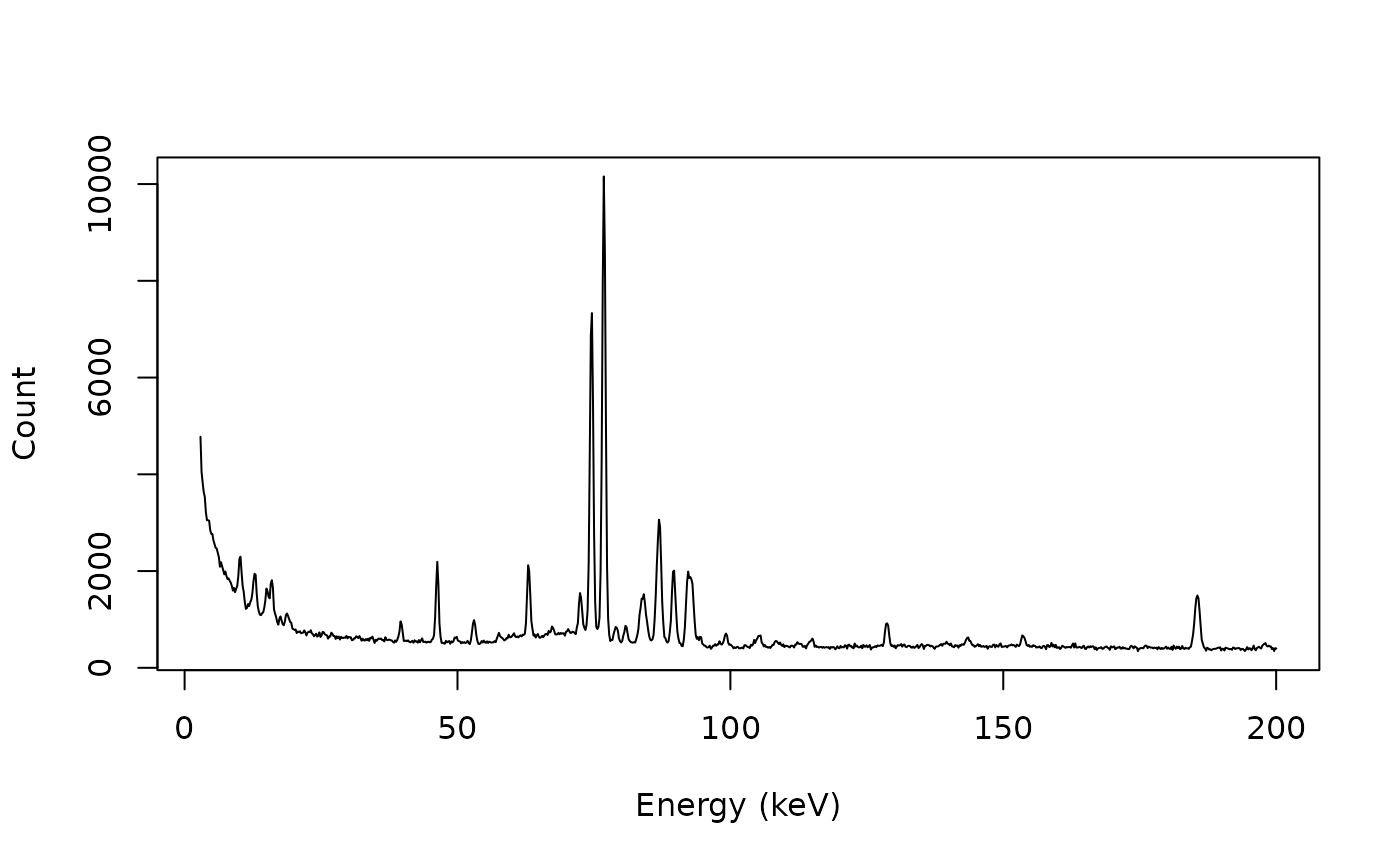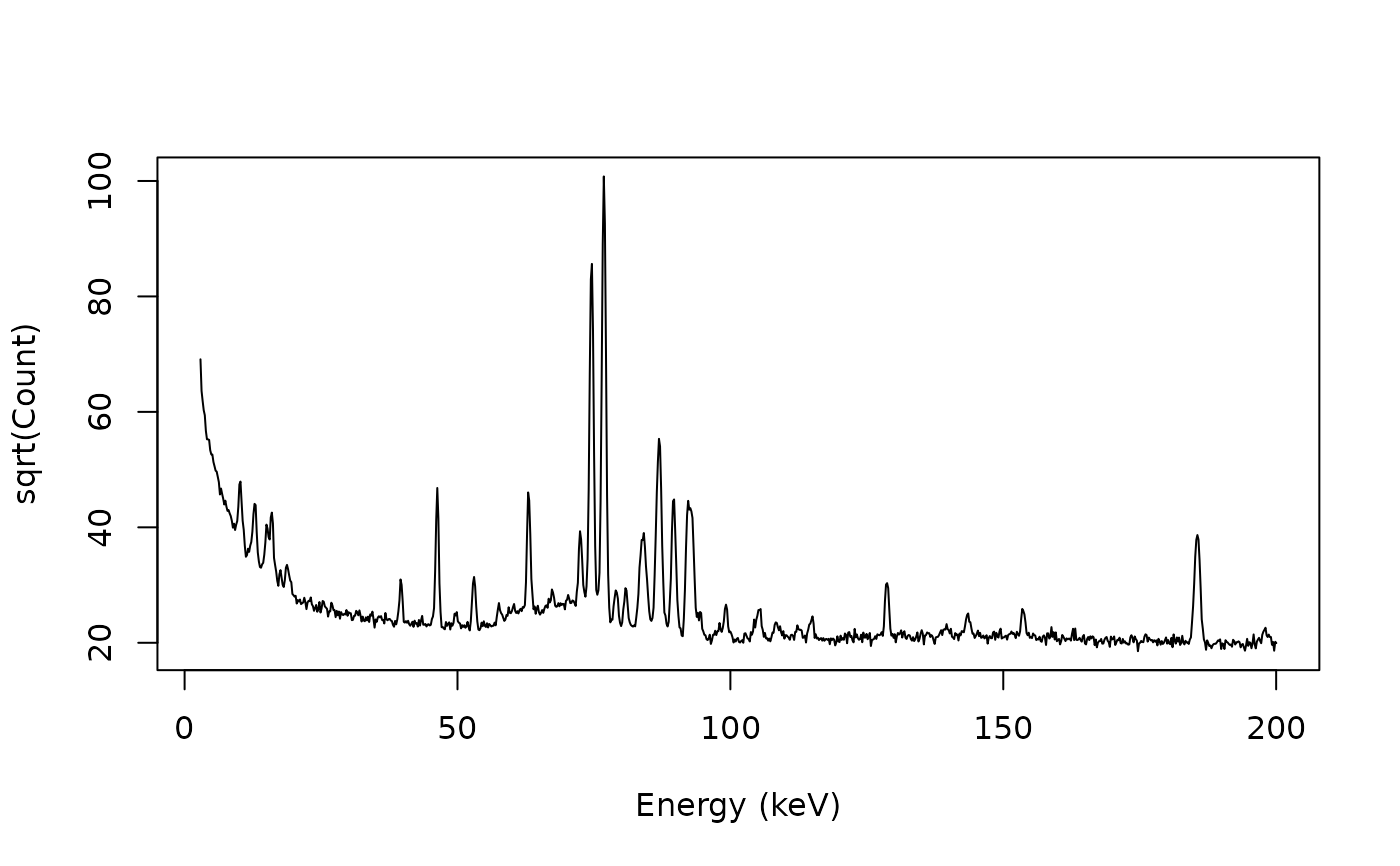Transform Intensities

## Usage

rescale_transform(x, y, ...)

# S4 method for numeric,numeric
rescale_transform(x, y, f, ...)

# S4 method for ANY,missing
rescale_transform(x, f, ...)

## Arguments

x, y

A numeric vector. If y is missing, an attempt is made to interpret x in a suitable way (see grDevices::xy.coords()).

...

Extra arguments to be passed to f.

f

A function that takes a numeric vector of intensities as argument and returns a numeric vector.

## Value

Returns a list with two components x and y.

## Details

Transformation of intensities can be used to improve the identification of peaks with a low signal-to-noise ratio.

Other normalization methods: rescale_area(), rescale_range(), rescale_total()

N. Frerebeau

## Examples

## gamma-ray spectrometry
data("BEGe")

## Subset from 2.75 to 200 keV
BEGe <- signal_select(BEGe, from = 3, to = 200)

## Plot spectrum
plot(BEGe, type = "l", xlab = "Energy (keV)", ylab = "Count")## Transform intensities
BEGe_trans <- rescale_transform(BEGe, f = sqrt)
plot(BEGe_trans, type = "l", xlab = "Energy (keV)", ylab = "sqrt(Count)")